# Piet Hein, Super Ellipses and Soma Cubes

### Contents

#### Piet Hein (1905-1996)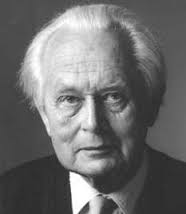Let's quote the entry about Piet Hein in David Darling's Enclopedia of Science, http://www.daviddarling.info/encyclopedia/H/Hein.html

An extraordinarily creative Danish mathematician, inventor, and poet who often wrote under the Old Norse pseudonym "Kumbel" meaning "tombstone." A direct descendant of the Dutch naval hero of the 16th century who had the same name, Piet Hein was born in Copenhagen and studied at the Institute for Theoretical Physics of the University of Copenhagen (later the Niels Bohr Institute) and the Technical University of Denmark.

[Piet Hein] is famed for his many mathematical games, including Hex, Tangloids, Polytaire, TacTix, and the Soma Cube, his advocacy of the superellipse curve in applications as diverse as city planning and furniture making, and his thousands of short, aphoristic poems called Grooks.

#### Grooks

This is one line from one of the Grooks.

      "Problems worthy
of attack
prove their worth
by hitting back!"
-- Piet Hein

#### Porcelain

My wife and I purchased one of these Piet Hein pocelain baking dishes years ago during a visit to Denmark. It's been a mainstay in our kitchen ever since. This photo is not from our kitchen, it's from a page at http://www.piethein.com featuring .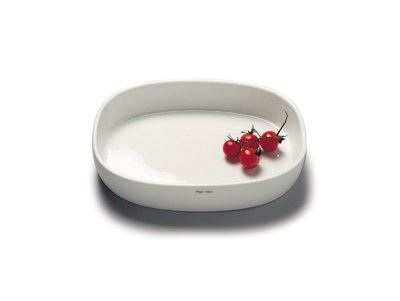#### Super Ellipses

Hein's design of the baking dish, as well as many other items, is based on what he called the superellipse, a curve that lies between old-fashioned ellipses and rectangles. The important parameter $p$ controls the roundness. Additional parameters $a$ and $b$ determine the aspect ratio. A superellipse with these parameters is the set of points $(x,y)$ satisfing

$$|\frac{x}{a}|^p + |\frac{y}{b}|^p = 1$$

If $a$ and $b$ are both equal to one, the equation is

$$|x|^p + |y|^p = 1$$

And if $p$ is equal to two it becomes

$$x^2 + y^2= 1$$

which defines a circle.

Here is the MATLAB definition.

   F = @(x,y) abs(x/a).^p + abs(y/b).^p - 1;


Let's vary p between 0.1 and 10 and make an animated gif, pausing briefly as we pass through p = 1 and p = 2.

   type make_superellipse_movie

    gif_frame('superellipse_movie.gif')
for p = [0.1 0.1 0.1:0.1:1 1 1 1:0.2:2 2 2 2:.5:10]
F = @(x,y) abs(x).^p + abs(y).^p - 1;
ezplot(F,[-1.2 1.2])
axis square
title(['|x|^p + |y|^p = 1, p = ',sprintf('%.1f',p)])
gif_frame
end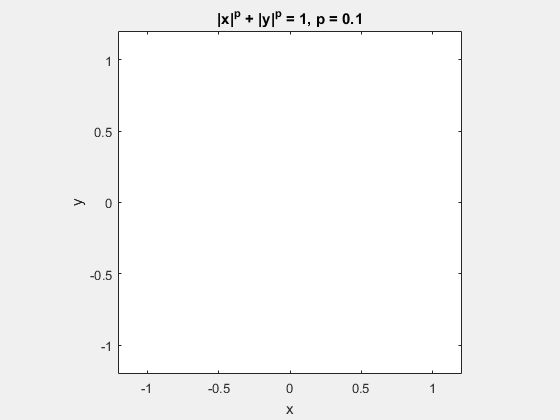All of Piet's designs have p = 2.5.

   p = 2.5;
a = 1.5;
F = @(x,y) abs(x/a).^p + abs(y).^p - 1;
ezplot(F,[-1.8 1.8])
axis equal
title(sprintf('Superellipse, p = %.1f',p))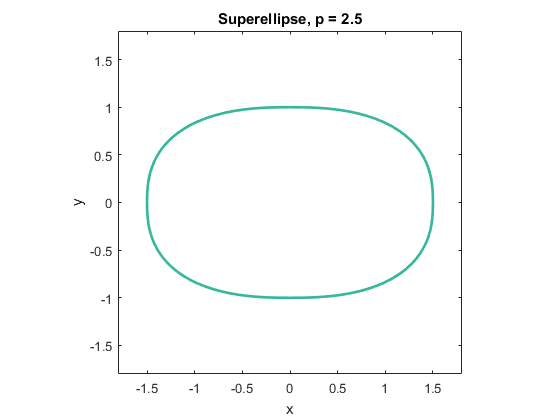Different scale factors on x and y make the shape rectangular and p greater than two makes it not exactly an ellipse.

#### The p-norm

I could have said that the superellipse is the unit ball in two dimensions of the vector p-norm,

$$||v||_p = \big( \sum_i |v_i]^p \big)^{1/p}$$

In MATLAB this is norm(v,p). In other words the function F starring in the first animated gif could have been defined by

   F = @(x,y) norm([x,y],p) - 1;


When p = 1 the unit "ball" becomes the unit diamond. And for p < 1 the quantity norm(v,p) is no longer actually a norm. It fails to satisfy the triangle inequality.

Homework: what is norm(v,p) for negative p, and when p = -Inf?

#### Soma Cube

Use the Images option in Google to search for all of the different images of "Soma Cube" available on the Internet. It's awesome.

Here is one of those images, available from http://mathcats.org/ideabank/geometrykits.html. This is all possible shapes with a reentrant corner that can be made out of three or four "cubelets". There are a total of 27 of those cubelets. The goal is to make a 3-by-3-by-3 larger cube.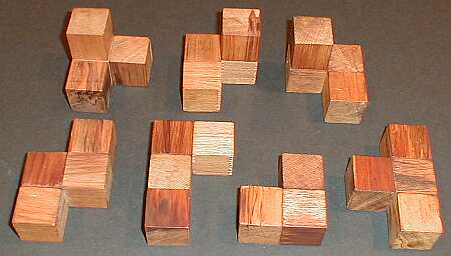My father's hobby was woodworking. He had a very nice shop. One year when I was a kid, he and I made 12 Soma Cube puzzle sets like this one to give to our family for Christmas. My set is now over 65 years old, but I still have it in my office at home.

#### Soma Cube demo

The following is the shortest code segment I've ever presented in over 2-1/2 years of Cleve's Corner blog. In the command window of your own copy of MATLAB you can enter.

soma


This launches an old demo written by Bill McKeeman. Click away, to your heart's content. In this animated gif, watch the solution number change . It's looping over only the first 12 out of the 240 possible puzzle solutions that McKeeman's algorithm finds.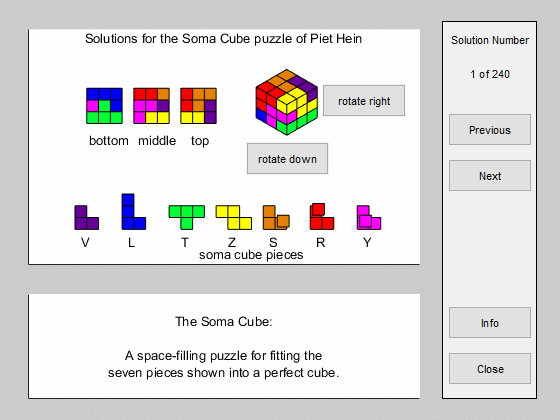McKeeman and I were buddies in grad school. We spent too many hours perfecting our Some Cube skills. In fact, I can still put the puzzle together behind my back.

Published with MATLAB® R2015b

|